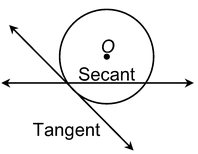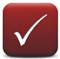# Circle

## Circles of Class 10

A circle is the locus of a point which moves in a plane in such a way that its distance from a fixed point remains constant.

### SECANT:

A line, which intersects a circle in two distinct points, is called a secant.### TANGENT:

A line meeting a circle only in one point is called a tangent to the circle at that point.

The point at which the tangent line meets the circle is called the point of contact.

### LENGTH OF TANGENT:

The length of the line segment of the tangent between a given point and the given point of contact with the circle is called the length of the tangent from the point to the circle.There is no tangent passing through a point lying inside the circle. There is one and only one tangent passing through a point lying on a circle. There are exactly two tangents through a point lying outside a circle.

Do solve NCERT text book for class 10 maths with the help of Physics Wallah NCERT solutions for class 10 maths.